5.8. Analysis of Space Contortion and Black Hole Formation

Newton’s law of gravitation (1687) encouraged the development of the astronomical ideas , , . First Michell (1783), then Laplace (1786) predicted the possibility of existence of the stars with a strong enough gravitational field that can retard the light photons, that’s why such stars become invisible . Later, they were called the black holes. Einstein’s general theory of relativity predicts the existence of the black holes as well .

In 1916, Karl Schwarzschild, the German astronomer and physicist, offers a formula (89) for the calculation of the gravitational radius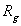of the black hole, which originates from the law of classical mechanics . Since then this formula has been used in the astronomic calculations, and the gravitational radius is called Schwarzschild’s radius: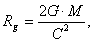(89)

where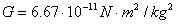is gravitational constant,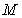is mass of the star;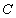is velocity of light.

It is known that as the photon wave length is reduced (from the infrared to gamma range), its energy is increased by a factor of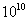. The possibility of the photon to overcome the gravitational force is increased in the same degree, but it is not taken into account in the formula (89). We have every reason to believe that a mistake has been made when the formula has been derived. What is its kernel? The formula (89) has been derived in the following way. The mathematical relation of the law of gravitation was been taken as a basis , :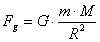,                                                                                 (90)

here: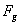is gravitational force;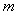is the photon mass;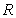is the distance between the centres of the masses of the bodies, which form the gravitation.

In order to find the gravitational radius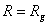of a star, by which its gravitational field retards light, it is necessary to find an equation between the gravitational force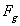and the force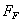, which moves the photon. But it is not simple to do it when there is no information concerning the electromagnetic structure of the photon. That’s why the idea of the equality between the energy of the photon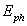and the potential energy of the gravitational filed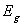. If we assume that the gravitational force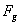performs the work at a distance, which is equal to the gravitational radius, this work will be equal to :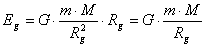.                                                       (91)

The bond between the energy of the photon,  its wave length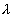, oscillation frequency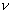and velocityis determined by the dependencies , , :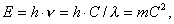(92)

where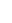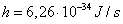is the Planck’s constant;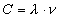.

Then it has been assumed that the photon will move in the gravitational field of the star with velocity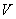, that’s why its kinetic energy should be determined by the relation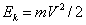. When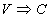, we’ll have: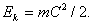(93)

It means that the gravitational field of the star will retard the photon when its potential energy (91) and kinetic energy of the photon (93) are equal, i.e.: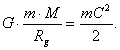(94)

We get the formula (89) for the calculation of the gravitational radius, which has been suggested by K. Schwarzschild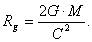(95)

The investigations carried out by us show that velocity of the centre of mass of the photon is changed in the interval of its wave length in such a way that its mean remain constant, and is equal to velocity of light. We can determine the force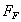, which moves the photon, in the first approximation by means of division of its energy by the wave length , .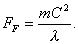(96)

If we equate the gravitational force (90) and the force moving the photon (96), we’ll have: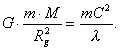(97)

It is clear that in order to convert the equation of the forces (97) into the equation of energies (94) it is necessary to reduce the denominator of the left-hand part by the gravitation radiusand to reduce the denominator of the right-hand side by the wave-length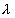of the photon. Actually it means that  it is necessary to equate the gravitation radius to the wave length of the photon. Certainly, it is impossible to do it, but Schwarzschild did it, and his followers did not notice this error. Thus, we have found the reason of the absence of the wave-length of the photon in the formula (89) in order to calculate the gravitational radius of the black hole. It is the result of Schwarzschild’s mistake made by him when he has derived this formula.

It means that in order to determine the gravitational radius of the black hole it is necessary to use the equality between the gravitational force and the force, which moves the photon, but not the equality of energies.

Photon moving force (87) can be written in the following way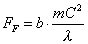,                                                                              (98)

where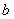is coefficient, which value depends on acceleration used during calculation.

For maximal overall acceleration (86)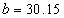; for maximal tangential acceleration (82)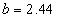; for maximal value of projection of overall acceleration on axis OX coinciding with the direction of centroidal motion of the photon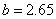. In our previous publications  we have assumed that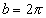. It corresponds to maximal overall acceleration of the point of conventional circumference of radius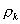. As the value of the above-mentioned coefficient exerts insignificant influence on the value of acceleration of the centre of mass of the photon, let us accept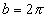for the case being considered by us.

If force (98)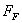, which moves the photon, is equal to gravitational force (90), gravitational force of the gravitational field retards the photon.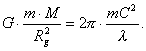(99)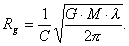(100)

Thus, we have got the formula for the calculation of the gravitational radius of the black hole, which takes into consideration the wave-length of the electromagnetic radiation.

Then the force, which moves the light photon with the wavelength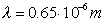with velocity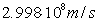, will be :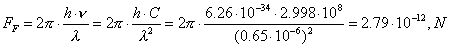(101)

Assuming that mass  of the Sun is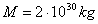, the radius of the Sun is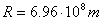, the gravitational constantand designating mass of the photon via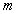, we’ll determine force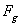of gravitation of the Sun, which influences the passing photon, according to the formula  , :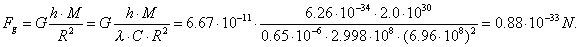(102)

When the photon passes the Sun, tangent of the angle of deviation of the photon from the straight-line motion will be equal to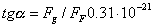.

If the photon the wavelength of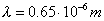passes the Sun along the straight line, which is parallel to the line connecting the centres of masses of the Sun and the Earth, the value of its deviation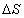from the straight-line motion in the vicinity of the Earth will be , :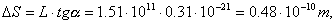(103)

where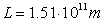is the distance from the Earth to the Sun.

At present time, science has no devices, which can register the value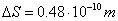.

Now gravitational radiusof the Sun, by which it becomes a black hole, is determined according to the formula (89), which does not take into account the wave-length of the photon , :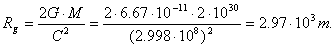(104)

Let us determine gravitational radii of the Sun for the infrared, light and gamma photons with the following wave lengths, respectively: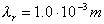,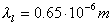и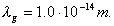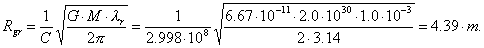(105)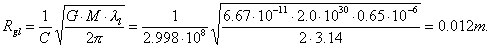(106)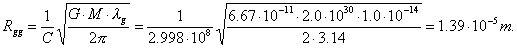(107)

Under the usual conditions, density r of the substance of the Sun is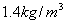. After compression, density of the substance of the Sun will depend on the gravitational radius, which is determined according to the formulas (104), (105), (106) and (107), respectively: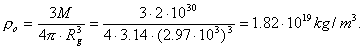(108)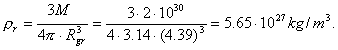(109)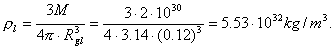(110)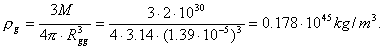(111)

Let us remind that density of nuclei of the atoms is determined by the value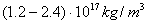.

It is clear that if the Sun is compressed to the gravitational radius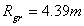(105), its gravitational field will retard only the radiation of the far infrared range of the spectrum. Electromagnetic radiation with smaller wave-length will penetrate easily. In order to retard the photons of all frequencies, the gravitational radius of the Sun should be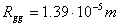(107); it is hardly possible, because in this case density of the substance of the Sun should be by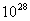greater than density of nuclei of the atoms , .

Thus, an error in the determination of the gravitational radius of the black hole according to the formula (89), which does not take into account the wave length of the electromagnetic radiation, is 108, but the astronomers do not know it yet , .

If the objects with such powerful gravitation, which retards electromagnetic radiation, exist in the nature, not all of them can be black. Their colours should be changed in compliance with the change of the colours of the photons, which cannot be retarded by these objects. The photons from the infrared range of the spectrum will be the first to be retarded; as the gravitational radius is reduced, the photons of the light, ultraviolet, roentgen and gamma ranges will be retarded. The hole becomes the black one only in such a case when the gravitational radius corresponds to the gamma photon with the minimal wave length.

5.9. Analysis of Michelson-Morley Experiment

The Russian scientist V.A. Atsyukovsky analysed rigorously the experimental data of the basis of Einstein’s theories of relativity and came to the following conclusion: “Analysis of the results of the experiments carried out by various investigators in order to check the provision of STR and GTR have proved that there are no experiments, in which positive and unambiguously interpreted results have been obtained confirming provisions and conclusions of theories of relativity of A. Einstein” .

This conclusion covers the most famous experiment: Michelson-Morley experiment. Please, pay attention to the fact that Michelson-Morley interferometer was stationary in relation to the Earth; it was light that moved. The authors thought that they would be lucky enough to register the influence of the Earth traverse speed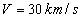in relation to the Sun on light fringe deviation. The calculation was carried out according to the formula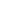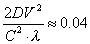(112)

Anticipated bias 0.04 of fringe was not registered. Somehow, the authors did not try to find the reason of disaccords between the theory and the experiment. Let us do it instead of them.

First of all, the photons have mass. It means that their behaviour in the gravity force field should not differ in this field from behaviour of other bodies, which have mass, for example, from behaviour of a flying plane. Why did the idea fail to strike them concerning the check the influence of the Earth rotation rate in relation to the Sun on a plane’s flight? Both the plane and the photon have mass, that’s why there should be no difference  in influence of their traverse speed in relation to the Sun in the field of the Earth gravity force on their behaviour. Such influence on the plane is so small that the devices fail to register it. The Earth rotation rate in relation to its axis influences the plane’s flight. This rate should exert the same influence on motion of light in the field of the Earth gravity force. In this case, we should substitute not the Earth traverse speed in relation to the Sun (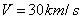in the above-mentioned formula, but the Earth surface speed (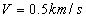) formed by its rotation in relation to its axis. Then the anticipated bias of fringe in Michelson-Morley experiment will be not 0.04, but significantly less: 0.00002. No wonder that Michelson-Morley device showed lack of fringe bias. Now we know the reason: it has no indispensable sensitivity (accuracy) .

What will happen if an experiment is carried out, in which a source of light and a device registering the fringe bias move (rotate) in the Earth gravitational field? In this case, the readings of the devices are compared when there is no rotation of the whole installation and during its rotation. It is clear that if there is no rotation of the installation, the principle of measurements will not differ from the principle of measurements in Michelson-Morley experiment, and the device will show no fringe bias. When the installation begins its rotation in the Earth gravitational field, the bias of the above-mentioned fringe should take place at once. It is explained by the fact that when light travels from the source to the receiver, the latter’s position is changed in the Earth gravitational field in relation to the source, and the device should register bias of the above-mentioned fringe.

Let us emphasize once again: the position of the source and the signal receiver  in Michelson-Morley experiment is not changed in relation to each other in the Earth gravitational field, and it is changed in the example described by us. This is the main difference of the above-mentioned experiments. The elementary logic being described is proved by Sagnayk’s experiment. The results of his experiment contradict to the readings of Michelson-Morley interferometer, and this fact is ignored by the relativists. It proves the fact that they are not interested in scientific truth .

We have given strong reasons of erroneousness of Einstein’s theories of relativity. That’s why one is tempted to ask the question: how should we accept the fact that his theories of relativity serve as a foundation of all achievements of physics in this century as the relativists think? It is very simple! All these achievements are the results of the efforts of the physics-experimenters who have carried out the experiments in the order not to check physical theories, but to get a result, which could be used for military purposes or in competition on purpose to work up a sales market for their products.

The theoreticians tried to find the explanation for these achievement and to substantiate them somehow, but these explanations proved to be proximate and superficial. The stereotype of thinking formed by erroneous Einstein’s theories and inexplicable insistence of his adherents in protection of these theories was a main retardation  in explanation of the deep principles of matter and creation.

History of science remembers no case when the scientific ideas of any great scientist of the past, for example, of I. Newton have been defended with the same fury as Einstein’s ideas of relativity are defended. Albert Einstein was much more critical to his scientific results than his adherents. Let us cite his opinion once more: “It seems to them that I look at the results of my life with a halcyon satisfaction. But everything is to the contrary if examined closely. There exists no concept, in relation to which I am sure that it will remain inviolable, and I am not convinced that I am on the right track.” (F. Hernek. Albert Einstein. M., 1966, page 16).

These words do credit for their author. History of science knows many “irrefutable ideas”, which have proved to be erroneous during further development of science.

The Foundations of Physchemistry of Microworld

Ó2003 Kanarev Ph. M.

Internet Version - http://book.physchemistry.innoplaza.net

<< Back to Physchemistry Book Index